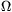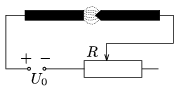Mathematical and Physical Journal
for High Schools
Issued by the MATFUND Foundation
 Already signed up? New to KöMaL?

#Problem P. 3930. (November 2006)

P. 3930. The circuit of a carbon arc lamp is shown in the figure (to ignite the lamp the carbon electrodes are touched together, then they are slowly drawn apart.) The voltage across the gap between the carbon rods as a function of the current can be given by the following formula:, where A and B are constants.

a) If the voltage of the battery U0. can be considered constant what is the resistance of the multiplier Rmax , that should be connected in series to ignite the lamp.

b) Find the stable current if the resistance of the multiplier is R<Rmax .

Data: A=30 V, B=20 W, U0=100 V, R=50.(5 pont)

Deadline expired on December 11, 2006.

### Statistics:

 36 students sent a solution. 5 points: Almási 270 Gábor András, Farkas Ádám László, Frigó Erzsébet, Gresits Iván, Szolnoki Lénárd, Tüzes Dániel. 4 points: Almasi Susann Melinda, Antal 888 Zoltán, Balogh Máté, Bogár 560 Péter, Drozdy András, Karsa Anita, Lovas Lia Izabella, Megyeri Balázs, Nagy 224 Csaba, Pásztor Árpád, Szőke Nóra, Varga Bonbien. 3 points: 15 students. 2 points: 1 student. 1 point: 2 students.

Problems in Physics of KöMaL, November 2006